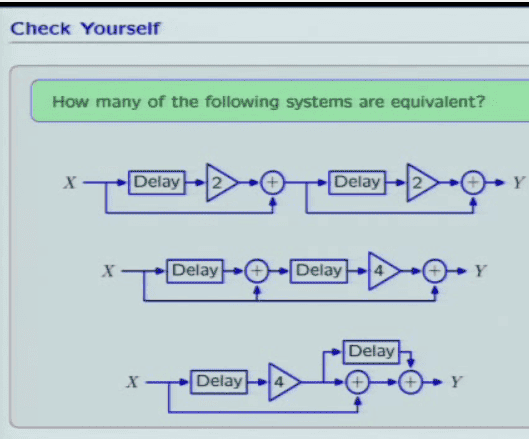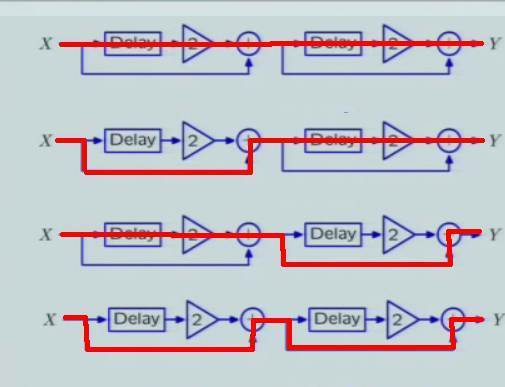# Equivalent Diagram- How does counting paths let us eyeball?

1. Homework Statement## The Attempt at a Solution

I wrote it out the operator expressions each by hand and got the same result but I don't understand how he is able to just eyeball it. I'm trying to develop some intuition.

a. To start, why is he counting distinct signal paths paths? What is the motivation to see why this information may be useful in letting us eyeball which block diagrams are teh same. He mentioned earlier in the video that this could be useful but I didn't understand.

b.

He says something about "making a sum" and seeing "how many of them have the same sum" What does that mean? He then looks for the paths with the biggest delay. I don't see why this info would help us other than to maybe to disprove two block diagrams are different.
^ See time stamped youtube vid

At the very end, he is able to conclude that the answer is 3 but I don't see how we have proof

NascentOxygen
Staff Emeritus
In (a) there are 4 paths: X; 4X2; 2X1; another 2X1
In (b) there are 3 paths: X; 4X2; 4X1
In (c) there are 3 paths: X; 4X1; 4X2

The output Y is the sum of these. The result: all are equivalent.

I have used X1 to denote X after one delay

BTW, I haven't looked at the videos.

rude man
Homework Helper
Gold Member
Call the output of every summing junction z
Then,for the 1st circuit,
z[n] = x[n] +2x[n-1]
and y[n] = z[n] + 2z[n-1]
then y[n] = x[n] + 2x[n-1] + 2x[n-1] + 4x[n-2]
= x[n] + 4x[n-1] + 4x[n-2]
You can proceed likewise for the 2nd and 3rd diagram to show y[n] is the same for all three.

Last edited:
In (a) there are 4 paths: X; 4X2; 2X1; another 2X1
In (b) there are 3 paths: X; 4X2; 4X1
In (c) there are 3 paths: X; 4X1; 4X2

The output Y is the sum of these. The result: all are equivalent.

I have used X1 to denote X after one delay

BTW, I haven't looked at the videos.

I have used X1 to denote X after one delay

So for example then, what does 4X2; 4X1 mean? Aren't there 9 paths because you have 4, 4, and 1? What is 4X2 for example? So it seems like based on your notation that would mean 4 times X after 2 delays in part a). Where does this 4 times X after two delays come from?

Is this his method of eyebaling?

NascentOxygen
Staff Emeritus
Those triangles with a number inside denote an amplifier (e.g., a voltage amplifier), they have no effect on the delay.
So I used 4X2 to denote X that has passed through two delays and has had its amplitude multiplied by 4. The order in which that has happened is irrelevant.

The circle with a cross in it represents a summer, its output is the sum of the inputs.

So the reason we know it is equivalent by eyeballing is that they all sum to 4X1; 4X2?

NascentOxygen
Staff Emeritus
So the reason we know it is equivalent by eyeballing is that they all sum to 4X1; 4X2?
Don't forget X. The outputs are all X + 4X1 + 4X2

NascentOxygen
Staff Emeritus